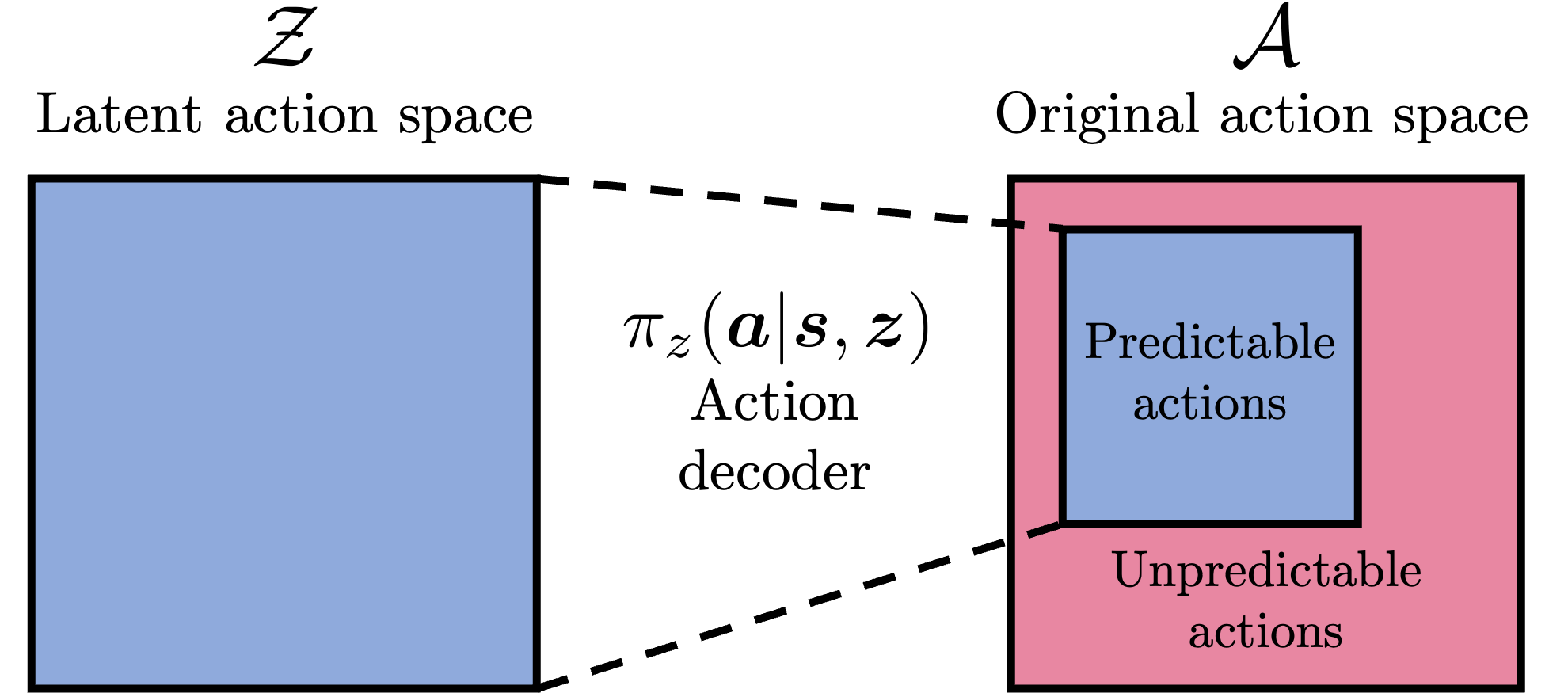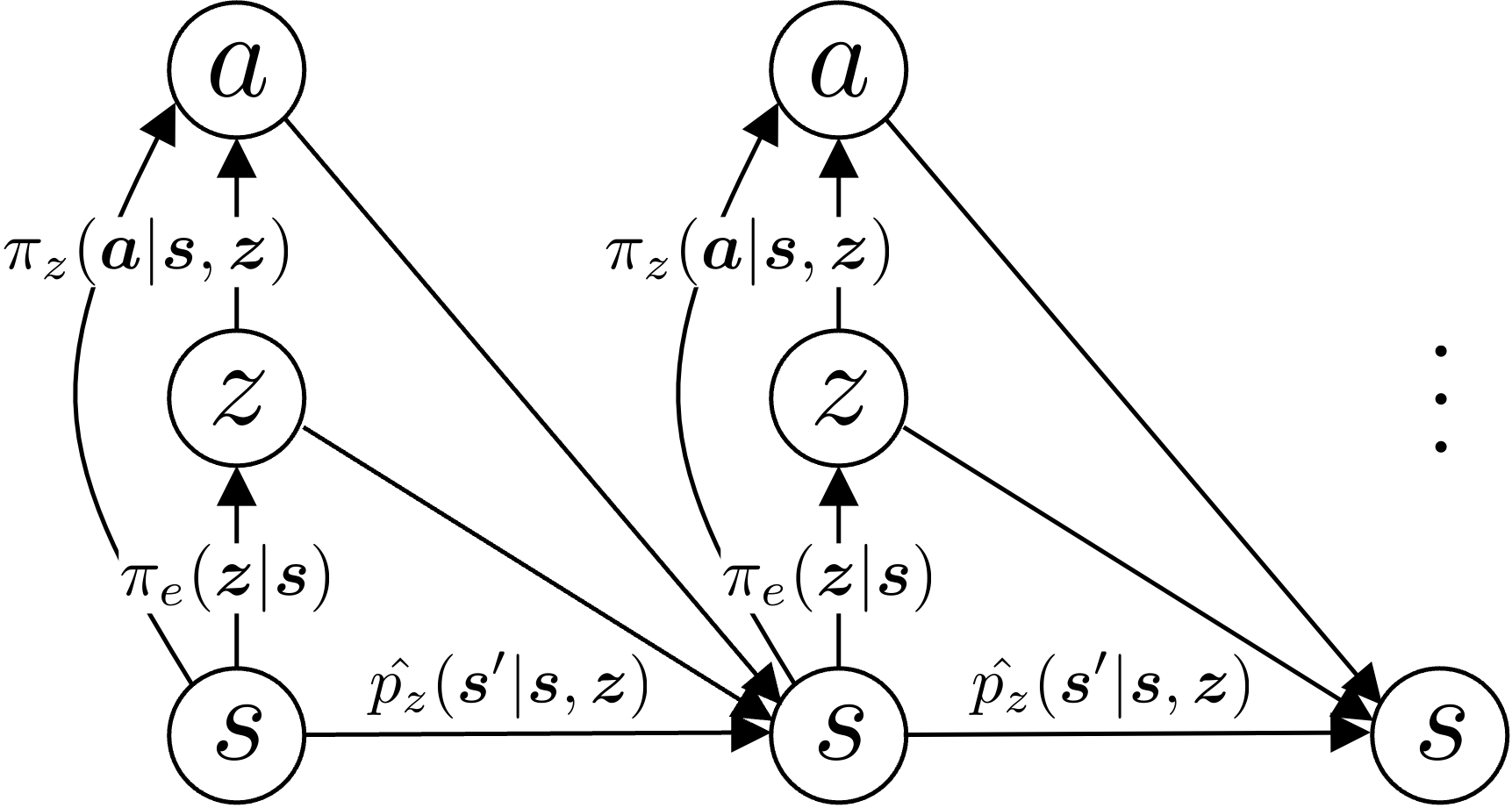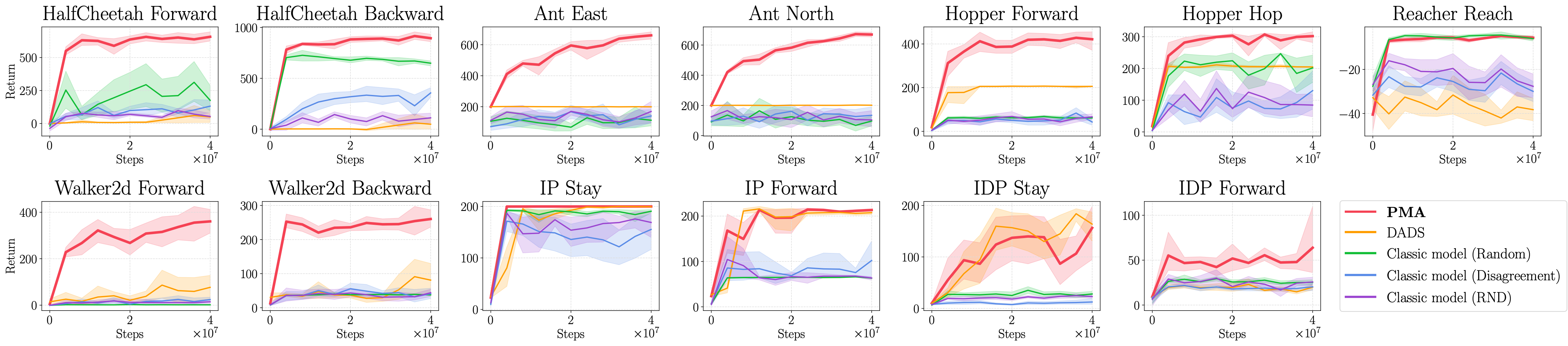## Abstract

A key component of model-based reinforcement learning (RL) is a dynamics model that predicts the outcomes of actions. Errors in this predictive model can degrade the performance of model-based controllers, and complex Markov decision processes (MDPs) can present exceptionally difficult prediction problems. To mitigate this issue, we propose predictable MDP abstraction (PMA): instead of training a predictive model on the original MDP, we train a model on a transformed MDP with a learned action space that only permits predictable, easy-to-model actions, while covering the original state-action space as much as possible. As a result, model learning becomes easier and more accurate, which allows robust, stable model-based planning or model-based RL. This transformation is learned in an unsupervised manner, before any task is specified by the user. Downstream tasks can then be solved with model-based control in a zero-shot fashion, without additional environment interactions. We theoretically analyze PMA and empirically demonstrate that PMA leads to significant improvements over prior unsupervised model-based RL approaches in a range of benchmark environments. Our code and videos are available at https://seohong.me/projects/pma/.

## Problem Setting and MotivationWe tackle the problem of unsupervised model-based RL, which consists of two phases. In the first unsupervised training phase, we aim to build a predictive model in a given MDP without knowing the tasks. In the subsequent testing phase, we are given multiple task rewards and aim to solve them only using the learned model without additional training; i.e., in a zero-shot manner. Hence, the goal is to build a model that best captures the environment so that we can later robustly employ the model to solve diverse tasks.

Most previous unsupervised model-based RL methods try to capture as many transitions $$p(\boldsymbol{s}'|\boldsymbol{s}, \boldsymbol{a})$$ as possible in the environment by running an exploration policy. However, accurately modeling all transitions is very challenging in complex environments (and even in completely deterministic MuJoCo environments, as shown in the videos below), and subsequent model-based controllers can exploit the errors in the learned model, leading to poor performance.

Our solution is to transform the original MDP into a predictable latent MDP, in which every transition is predictable. (Here, "predictable" also means that it is easy to model.) After the transformation, since our latent MDP is trained to be maximally predictable, there is little room for model exploitation. We can thus later robustly employ the learned latent predictive model to solve downstream tasks.

## Method OverviewThe main idea of PMA is to restrict the original action space so that it only permits predictable (e.g., easy-to-model) transitions. PMA has three learnable components. The action decoder policy $$\pi_z(\boldsymbol{a}|\boldsymbol{s}, \boldsymbol{z})$$ decodes latent actions into the original action space, effectively reparameterizing the action space in a lossy manner. The latent predictive model $$\hat{p}_z(\boldsymbol{s}'|\boldsymbol{s}, \boldsymbol{z})$$ predicts the next state in the latent MDP, which is jointly trained with the action decoder to make the learned MDP as predictable as possible. Finally, the exploration policy $$\pi_e(\boldsymbol{z}|\boldsymbol{s})$$ selects $$\boldsymbol{z}$$'s to train the PMA's components during the unsupervised training phase, maximizing the coverage in the original state space. As a result, the learned latent MDP becomes maximally predictable (i.e., having low model errors) while covering the original state-action space as much as possible.

Once we get a reward function in the testing phase, we replace the exploration policy with a task policy $$\pi(\boldsymbol{z}|\boldsymbol{s})$$, which aims to select latent actions to solve the downstream task. This task policy can be learned via model-based planning or model-based RL on top of our learned predictable MDP.

## The Objective

The desiderata of unsupervised predictable MDP abstraction are threefold. First, the latent actions in the transformed MDP should lead to predictable state transitions (i.e., predictability). Second, different latent actions should lead to different outcomes (i.e., diversity). Third, the transitions in the latent MDP should cover the original state-action space as much as possible (i.e., information gain). These three goals can be summarized into the following concise information-theoretic objective.

### \begin{aligned} &I(\boldsymbol{S}';(\boldsymbol{Z}, \boldsymbol{\Theta})|\boldsymbol{\mathcal{D}}) \\ = &-\underbrace{H(\boldsymbol{S}'|\boldsymbol{S},\boldsymbol{Z})}_{\text{predictability }} + \underbrace{H(\boldsymbol{S}'|\boldsymbol{S})}_{\text{diversity}} +\underbrace{H(\boldsymbol{\Theta}|\boldsymbol{\mathcal{D}}, \boldsymbol{Z}) -H(\boldsymbol{\Theta}|\boldsymbol{\mathcal{D}}, \boldsymbol{Z}, \boldsymbol{S}')}_{\text{information gain}} \end{aligned}

Here, $$\boldsymbol{S}$$ denotes the current state, $$\boldsymbol{S}'$$ denotes the next state, $$\boldsymbol{Z}$$ denotes the latent action, $$\boldsymbol{\Theta}$$ denotes the parameters of the latent predictive model, $$\boldsymbol{\mathcal{D}}$$ denotes the entire training dataset up to and including the current state. The first term maximizes predictability by reducing the entropy of the next state distribution $$p_z(\boldsymbol{s}'|\boldsymbol{s}, \boldsymbol{z})$$, making the latent MDP maximally predictable. The second term increases the entropy of the marginalized next state distribution, effectively making the resulting states from different $$\boldsymbol{z}$$'s different from one another. The third term minimizes epistemic uncertainty by maximizing information gain, the reduction in the uncertainty of the predictive model's parameters after knowing $$\boldsymbol{S}'$$. We refer to the paper for further details and its practical implementation.

With this objective as an intrinsic reward, we can optimize both the action decoder policy and exploration policy with RL. As a result, they will learn to produce the optimal $$\boldsymbol{z}$$'s and $$\boldsymbol{a}$$'s that in the long-term lead to maximal coverage of the original state space, while making the resulting latent MDP as predictable as possible.

## Examples of PMA

The videos below are some examples of PMA learned during the unsupervised training phase, showing model errors in the top left corners. PMA exhibits the lowest model error since it is trained to be maximally predictable.

## Zero-Shot Planning with PMA

During the testing phase, we can employ PMA's latent predictive model with any model-based planning or RL to solve downstream tasks in a zero-shot manner, i.e., without additional training or environment interactions. The videos below show some examples of zero-shot task-specific behaviors obtained by MPPI planning combined with a MOPO penalty. While PMA can robustly solve downstream tasks in a zero-shot fashion, classic models suffer from model exploitation due to high model errors.

## Quantitative Performance ComparisonWe quantitatively compare PMA with prior unsupervised model-based RL methods. Previous approaches typically pre-train a classic dynamics model of the form $$\hat{p}(\boldsymbol{s}'|\boldsymbol{s}, \boldsymbol{a})$$ using data gathered by some exploration policy. We consider three different exploration strategies: random actions ("Random"), disagreement-based exploration ("Disagreement"), random network distillation ("RND"). We also compare to DADS, a previous unsupervised skill discovery method that also learns a latent action dynamics model $$\hat{p}_z(\boldsymbol{s}'|\boldsymbol{s}, \boldsymbol{z})$$ but aims to find compact, temporally extended behaviors, rather than converting the original MDP into a more predictable one.

The figure above shows the periodic MPPI planning performances of PMA and the four previous methods on seven MuJoCo robotics environments with 13 diverse tasks, in which PMA achieves the best performance in most tasks. Especially, PMA is the only successful unsupervised model-based method in Ant, whose complex, contact-rich dynamics make it difficult for classic models to succeed because erroneous model predictions often result in the agent flipping over. We refer to the paper for more results and analyses.

The website template was borrowed from Michaël Gharbi and Jon Barron.